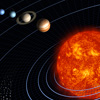Is the Universe simple or complex? Part II

Faye Kilburn

In search for an answer to this question the first part of this article led us to Occam's razor and, rather surprisingly, we ended up thinking about God. But is there a more objective assessment of simplicity and complexity?

Mathematical complexity

In 1963, Soviet mathematician Andrey Nikolaevich Kolmogorov formulated what is now known as Kolmogorov complexity to describe the mathematical complexity of an object. His idea, which was independently co-discovered by Argentine-American mathematician and computer scientist Gregory John Chaitin, says that the complexity of an object such as a piece of text is a measure of the computational resources needed to specify it. So if a 10 megapixels photo takes up 10MB — but you can store it as a compressed image in 300KB — then 300KB is the overall complexity of the photo (you can find out more in this Plus article). But is it possible to apply this simple description of complexity to the Universe?

Max Tegmark, a theoretical physicist at the Massachusetts Institute of Technology certainly thinks so, though he believes that scientists have a tendency to see complexity where there is none. For example, if we start with something familiar like our solar system, how many bits of information do you need to describe everything? There are eight planets in our solar system, so presumably the number eight needs to be part of the description. But since we know that there are other solar systems with other numbers of planets, Tegmark says the number eight does not in fact tell us anything fundamental about reality.Max Tegmark

Taking this to its logical conclusion, Tegmark argues that even the constants of nature, such as the gravitational constant or the speed of light, might also be illusory complexities, taking different values in different universes. If this is the case, all that is left is the mathematical framework of the theory of everything. "So the answer to the question 'how mathematically complex is the Universe?' would be, however complex the maths is. "Maybe it will take ages to write the maths, maybe you can fit it on a T-shirt – for now, we just don't know," he says.

In fact, Tegmark has one last simplification up his sleeve, though it's a controversial one. In his mathematical Universe hypothesis, Tegmark states that physical existence and mathematical existence are ultimately the same thing so that anything that exists mathematically also exists physically. Therefore, if string theory predicts more than one Universe, then those mathematical universes will correspond to physically real universes. So, all possible universes exist.

In order to describe one region (such as our Universe) in this infinite space of multiple universes, Tegmark says complicated details about how every atom started out must be provided, which is a huge amount of information. However, if you want to describe all possible infinite space, then it's easy because every possible event happens somewhere. "What's fun about this in the context the question 'is the Universe simple or complex?', is that it gives the most extreme answer of all. What's the complexity of nature of the whole of physical reality? I would say zero, because there are no physical parameters you need to specify and no equations you need to specify either. They all exist."

Too complex for our tiny little minds?

In our efforts to get to the bottom of the question "is the Universe simple or complex?," we've come full circle from intuitive ideas of physical complexity to Tegmark's hypothesis of elegant mathematical simplicity. Some academics believe that M-theory is the simplest possible explanation of the Universe whilst others such as Swinburne hold that God is simpler.

In Terry Pratchett's short story Death and what comes next Death proposes the following to a dying philosopher; "You are nothing more than a lucky species of ape that is trying to understand the complexities of creation via a language that evolved in order to tell one another where the ripe fruit was." It begs the question, are we capable of understanding these complexities? And can we make objective assessments about simplicity?

In his recent paper, Constructor theory, David Deutsch argues that our ideas of simplicity depend on our background knowledge; for example, we might judge calculus as fairly complex if we didn't know how to count. According to Deutsch, this background knowledge which informs our assessments of simplicity depends on our nature which ultimately depends on laws of physics. This means that some of the simplicity we ascribe to the laws of physics, might, to some degree depend on them. Deutsch calls it the mathematician's misconception. "What the rules of logical inference are, and hence what constitutes a proof, is not independent of the laws of physics," he says.

In this view, we are in no position to make judgements about the complexity of those nine million known species of life on Earth, 500 billion planets in the Milky Way, and 1078 atoms in the visible universe. We just aren’t capable of being objective. Similarly, our assessments as to whether one theory is simpler than other, and whether the world is more or less complex if it includes God are moot. The question "is the Universe simple or complex?" could be well beyond our ability to answer. Of course, that won't stop us from trying.